# Games

Most of the items in Political Games look at either “normal form games” (or “games in strategic form”) or “extensive form games.” Normal form games are games in which you specify three things: i) a set of players, ii) actions available for each, and iii) preferences over outcomes. Extensive form games add a temporal dimension which lets you model important strategic features like credibiity and time consistency.

In the `hop` package there are a suite a functions to model both types of game.

Simple normal form games can be illustrated nicely with payoff matrices — figures that represent these three items in a direct way. The payoff matrices in the book are all generated using a simple R function `gt_bimatrix` from the `hop` R package that takes a matrix of numbers as input and spits out a nice looking payoff matrix, indicating the pure strategy Nash equilibriums to boot.

A few other functions in the package can be used to examine the strategic logic of these games.

• With `br_graph` You can plot the utility of differet actions given probabilisic actions by opponents and plot best response functions in probability space.
• With `gt_brcplot` you can do the same thing for a continuous action space and identify equilibrium sets
• With `gt_coase` or `gt_nbs` you can plot the bargaining possibilities induced by a normal form game, and figure out the possible bargaining outcomes under the assumption of transferable utility.

For extensive form games the key function is `gt_tree` which can be used to plot game trees and find solutions to simple games.

Follow the buttons below for more.

## Getting Going

First open R or Rstudio and install the hop package from github (you will need to have devtools installed to do this, so perhaps you will need to `install.packages("devtools")`):

``devtools::install_github("macartan/hop")``

``library(hop)``

## Payoff matrices

The `gt_bimatrix` takes a payoff matrix and turns it into a nice figure. It can also mark best response functions as arrows and mark the (pure strategy) equilibriums as a star.

### Games with two players and two strategy options

The most basic games have just two strategy options for each player.

The prisoner’s dilemma matrix for example looks like this:

``PD <- matrix(c(2,4,1,3),2,2)``

Once defined you can plot it like this:

``gt_bimatrix(PD, nash = FALSE, arrow1 = FALSE,  tscale = .75)``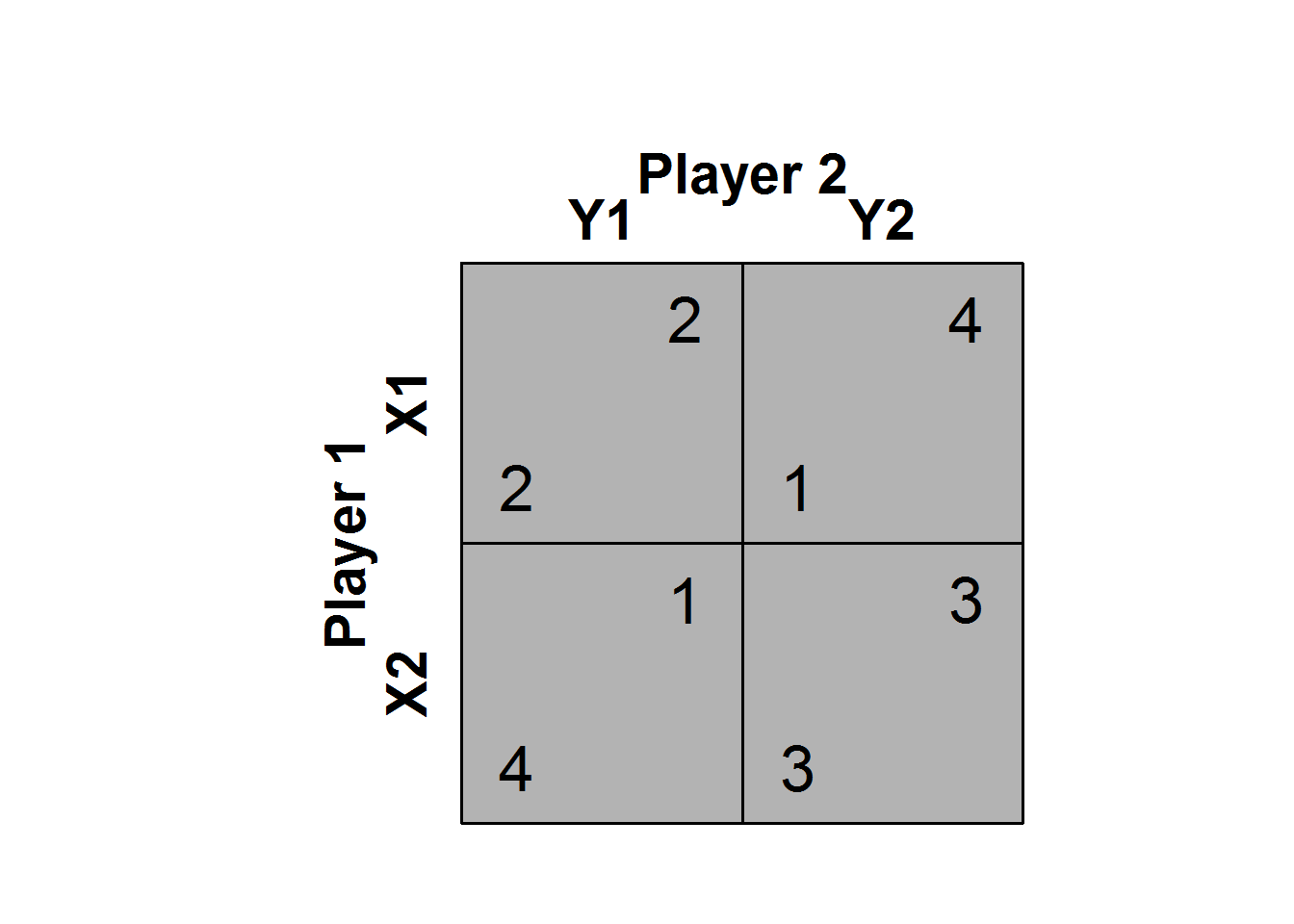And make it nicer like this:

``gt_bimatrix(PD, nash = FALSE, arrow1 = FALSE, P1 = "Jack", P2 = "Jill",  labels1=c("Cooperate","Defect"), srt1=90, tscale = .5)``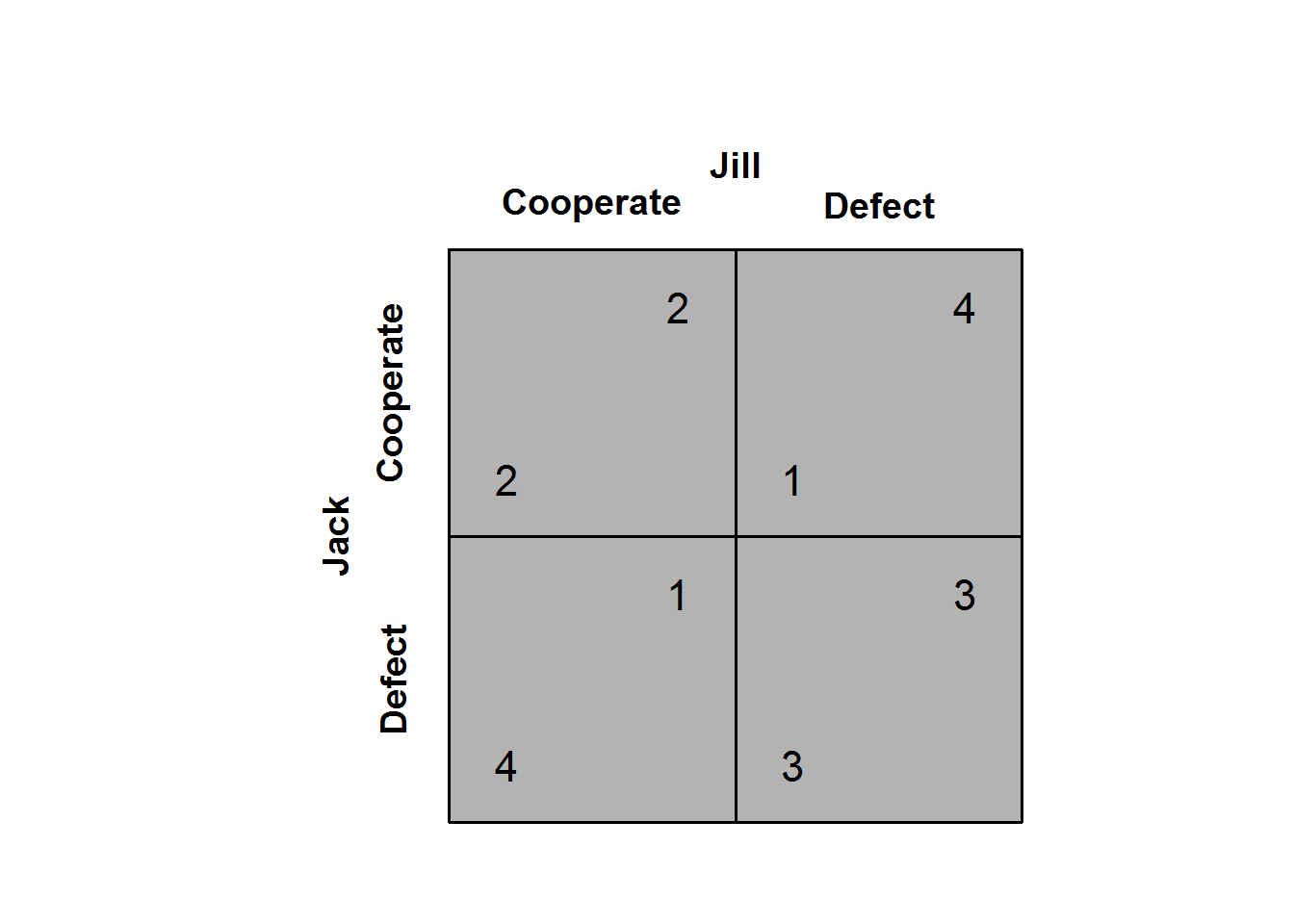Or go for a more conservative style using various options like this:

``````gt_bimatrix(matrix(c(1,0,0,0), 2, 2),
labels1 = c("U","D"), labels2 = c("L","R"),
pty = "m", matrixfill=NULL, nash = FALSE, arrow1= FALSE,
asp = .45, tfont = "serif", tscale = .7)``````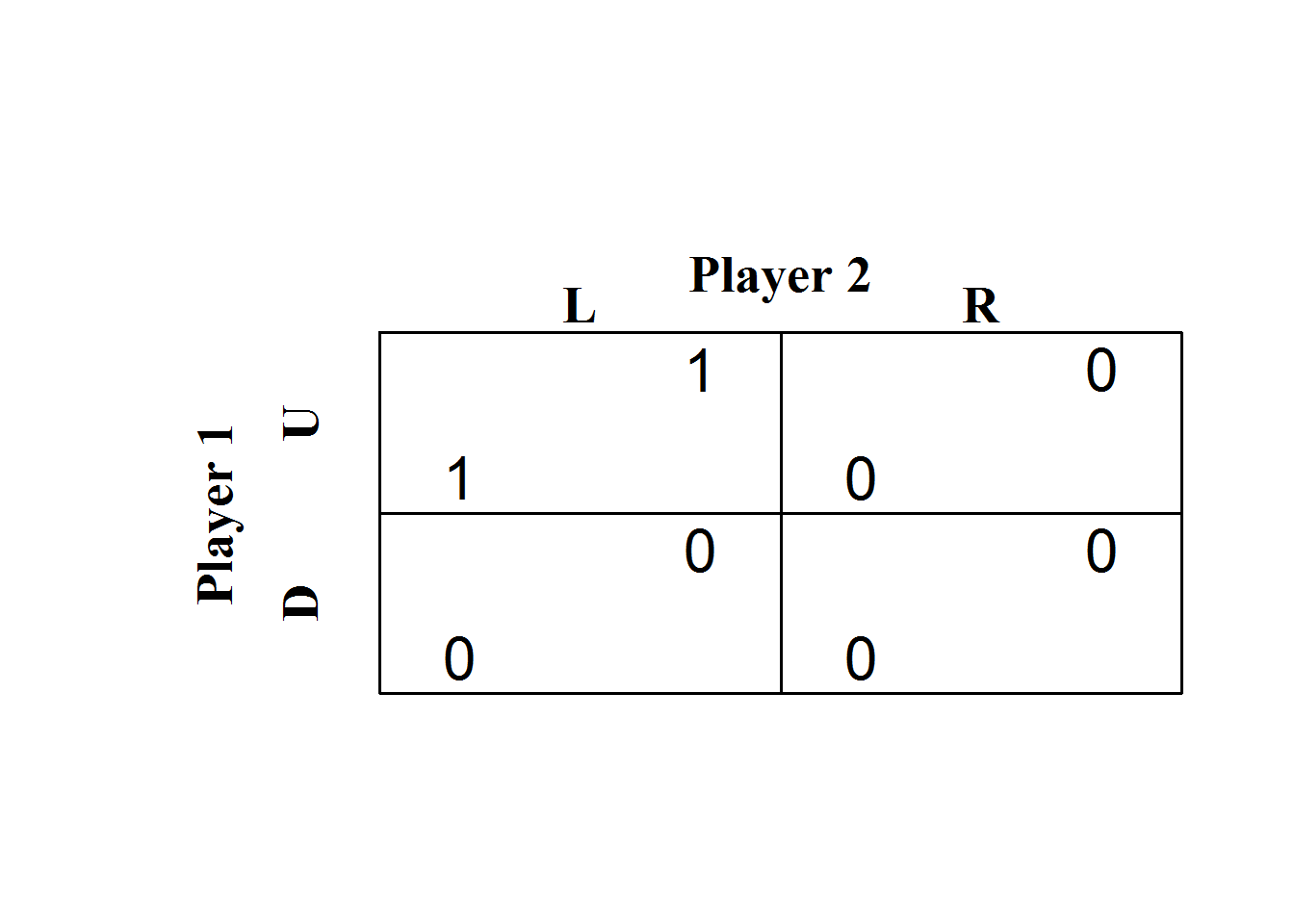You can also add in best response arrows that show the incentives to change strategies; like this:

``gt_bimatrix(PD, nash = FALSE, arrow1 = TRUE, P1 = "Jack", P2 = "Jill",  labels1=c("Cooperate","Defect"), srt1=90, tscale = .5)``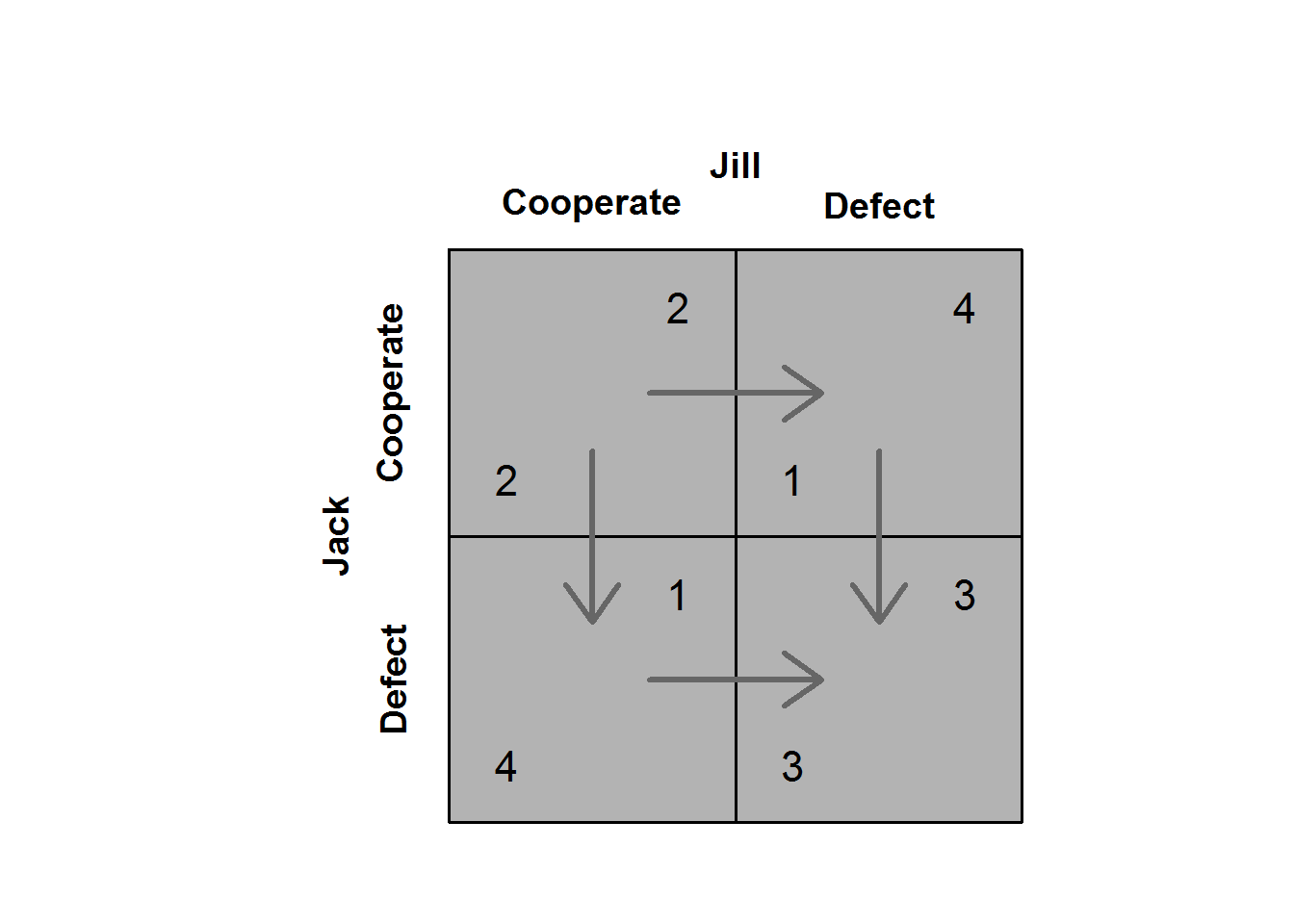And add in a star to mark the Nash equilibria:

``gt_bimatrix(PD, P1 = "Jack", P2 = "Jill",  labels1=c("Cooperate","Defect"), srt1=90, tscale = .5)``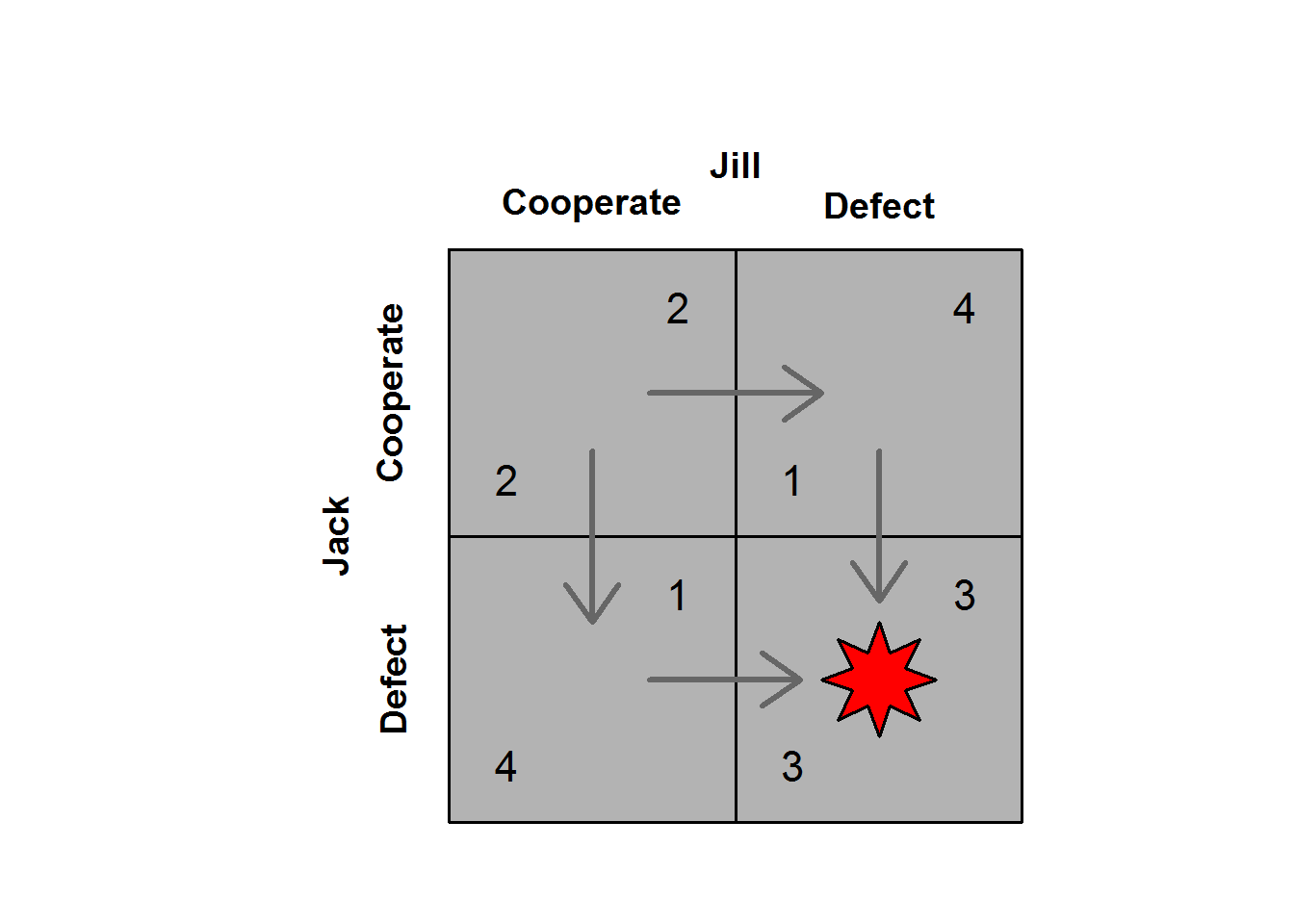Here is a comparison of a set of classic games:

``````par(mfrow=c(2,2))
gt_bimatrix(X = matrix(c(2,4,1,3),2,2), tscale = .4, width1 = 1, alength = .15, main = "PD")
gt_bimatrix(X = matrix(c(3,4,2,1),2,2), tscale = .4, width1 = 1, alength = .15, , main = "Chicken")
gt_bimatrix(X = matrix(c(4,2,1,3),2,2), tscale = .4, width1 = 1, alength = .15, , main = "Assurance")
gt_bimatrix(X = matrix(c(1,4,3,2),2,2), Y = matrix(c(2,3,4,1),2,2),
tscale = .4, width1 = 1, alength = .15, ,  labels1 = c("Boxing", "Ballet"), srt1=90,
main = "Battle of the Sexes")``````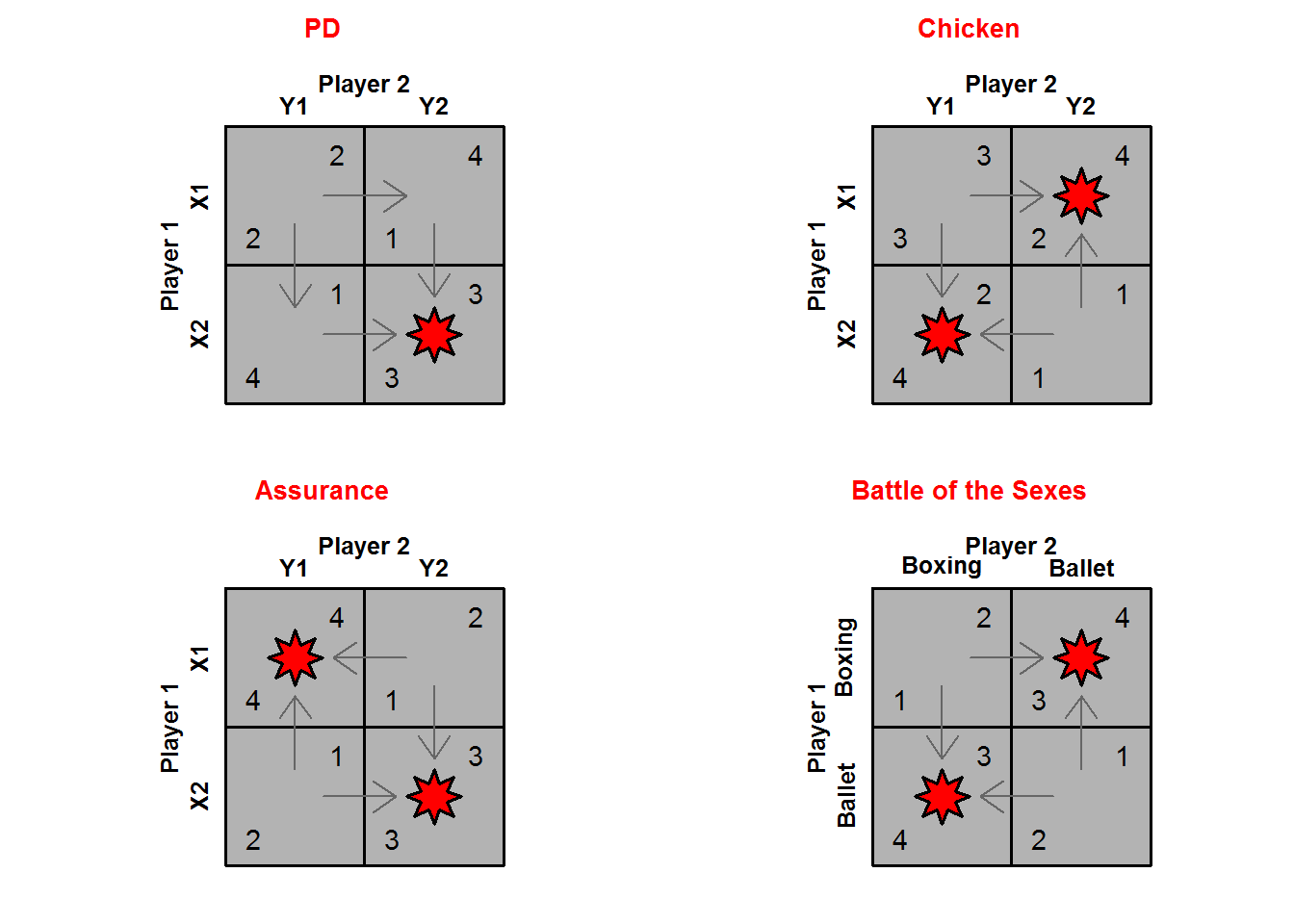### Two players but multiple strategies

Extending to games with multiple actions for two players is straightforward and there is no requirement that both players have the same options available to them. (Increasing the number of players is trickier than increasing the number of options)

In this example Jill has five options and Jack has just two. PLot the payoff matrix and you see that there are two pure strategy Nash equilibria.

``````Jack <- matrix(10*c(1:10) - (1:10)^2,2)
Jill <- matrix(10*c(1:10) - (1:10)^2,2)
gt_bimatrix(X=Jack, Y=Jill, P1 = "Jack", P2 = "Jill",  tscale = .5, width1 = 1.5, alength = .15, )``````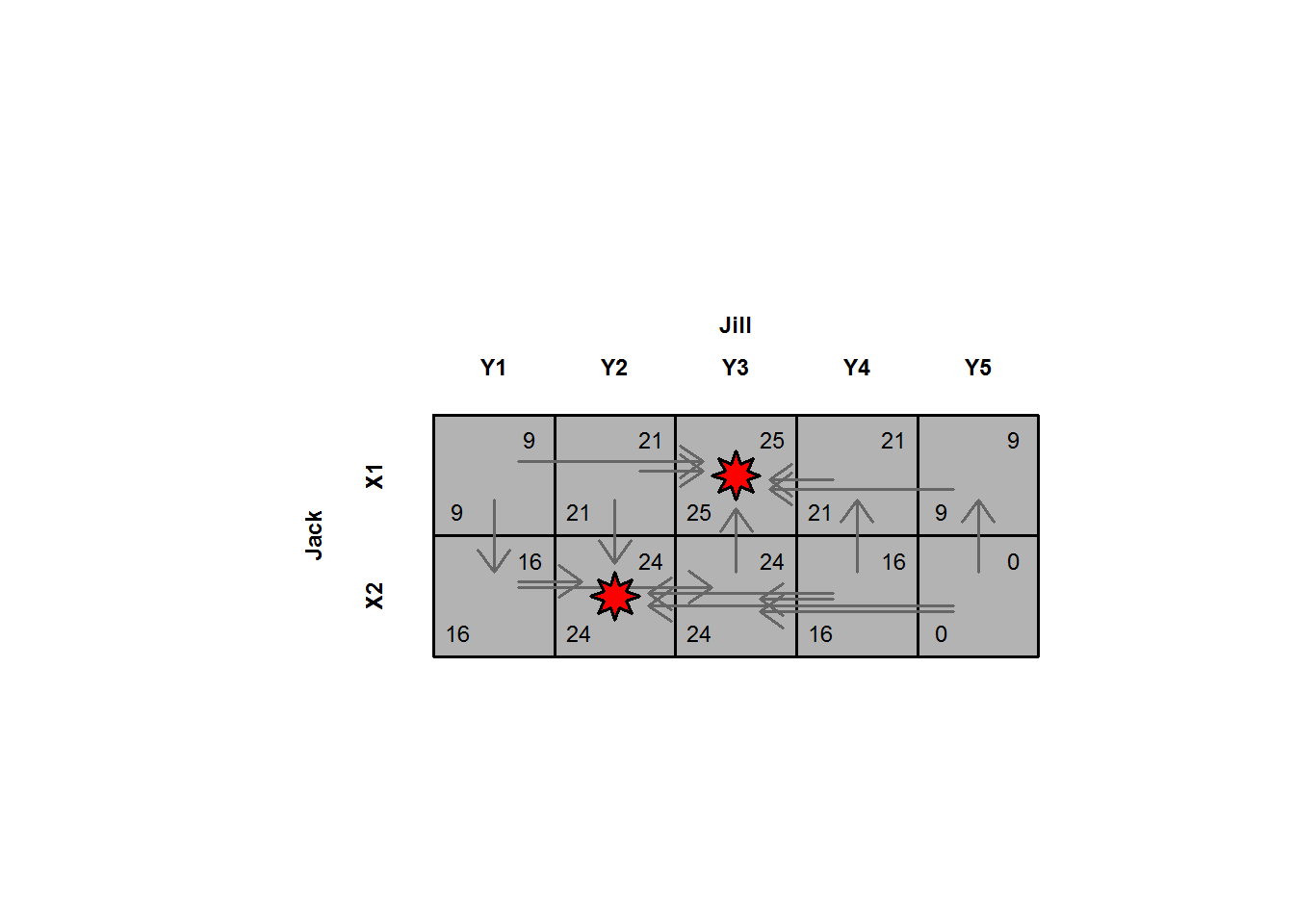## Best responses

Many of the canonical games also have mixed strategy equilibria. Thes ecan be identified by looking at the payoffs to different actions conditional on different probabilities with which your opponent plays their strategies.

Lets illustrate with the chicken game. First here is the payoff matrix:

``````Chicken <- matrix(c(3,5,2,1),2,2)
gt_bimatrix(Chicken, P1 = "Jack", P2 = "Jill", labels1 = c("C","D"), tscale = .75)``````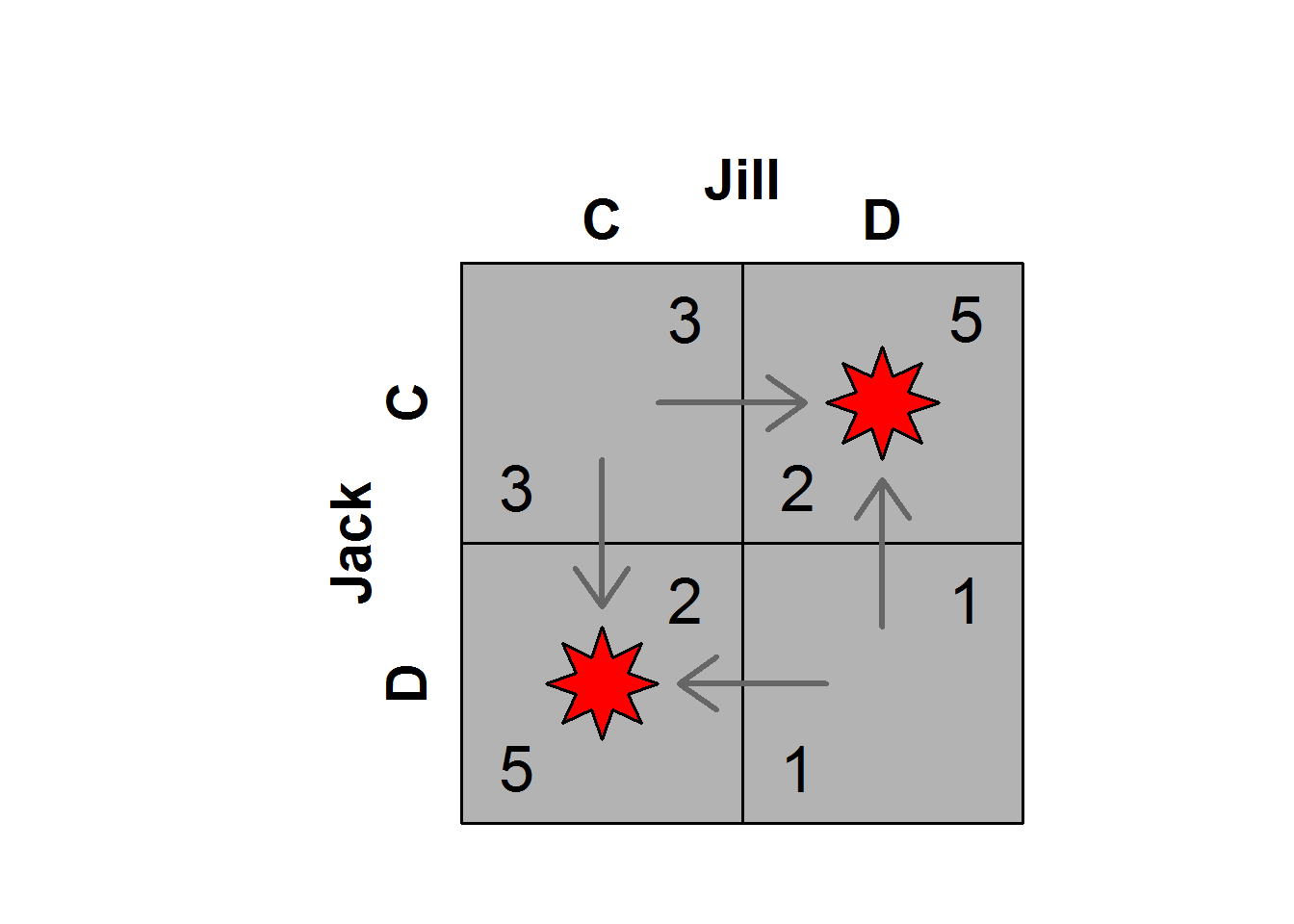Note that this particular game of chicken has joint gains maximized when only one side cooperates.

We can also represent the utilities for both players as a function of the probability with which Jack plays “C”:

``````par(mfrow = c(2,1))
gt_brgraph(Chicken, P1 = "Jack", P2 = "Jill", br = FALSE, util = TRUE, u1 = FALSE)
gt_brgraph(Chicken, P1 = "Jack", P2 = "Jill", br = FALSE, util = TRUE, u2 = FALSE)``````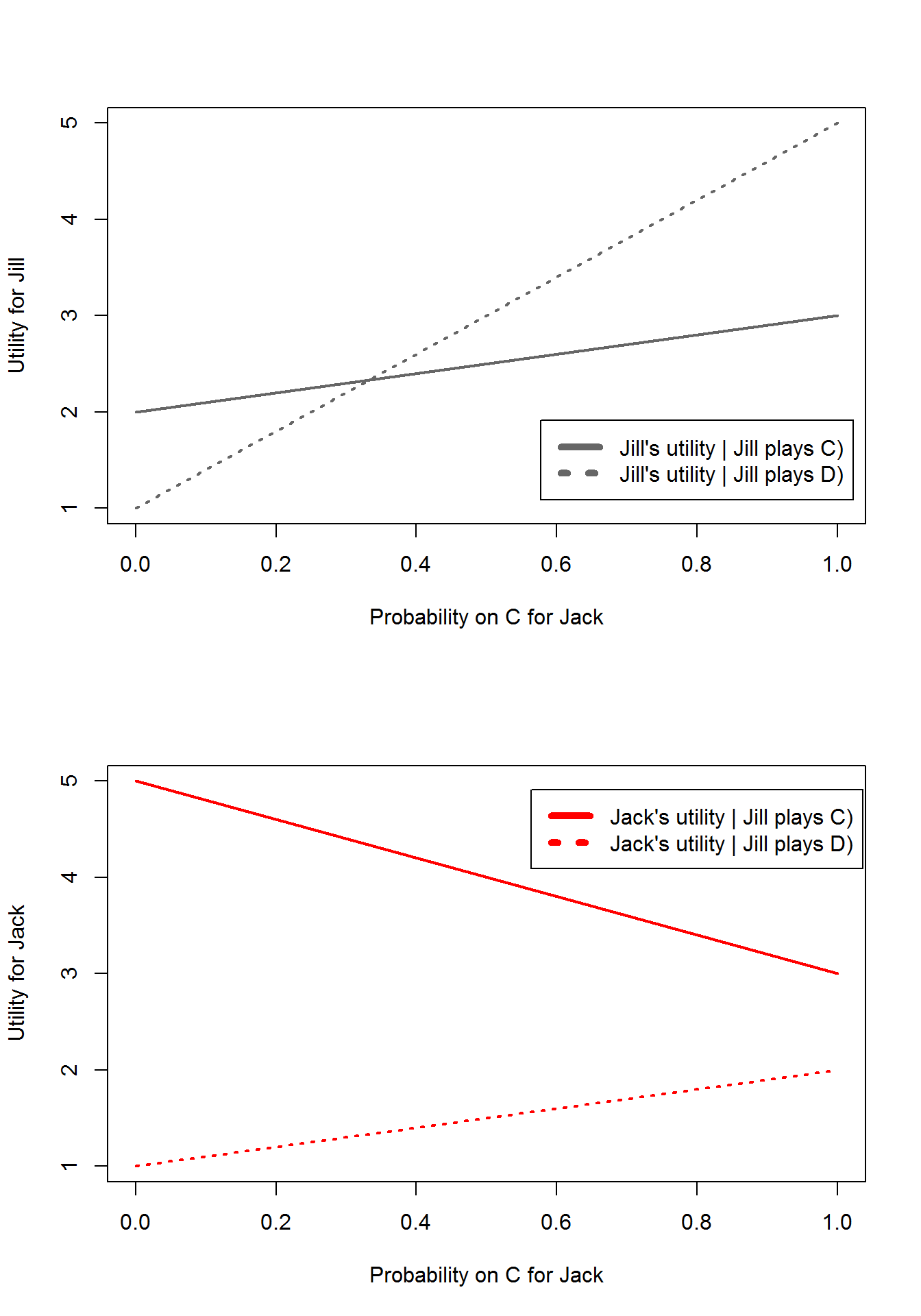You can highlight Jill’s best responses to any action by Jack like this:

``gt_brgraph(Chicken, P1 = "Jack", P2 = "Jill", br = FALSE, util = TRUE, cthighlight = TRUE, u1 = FALSE)``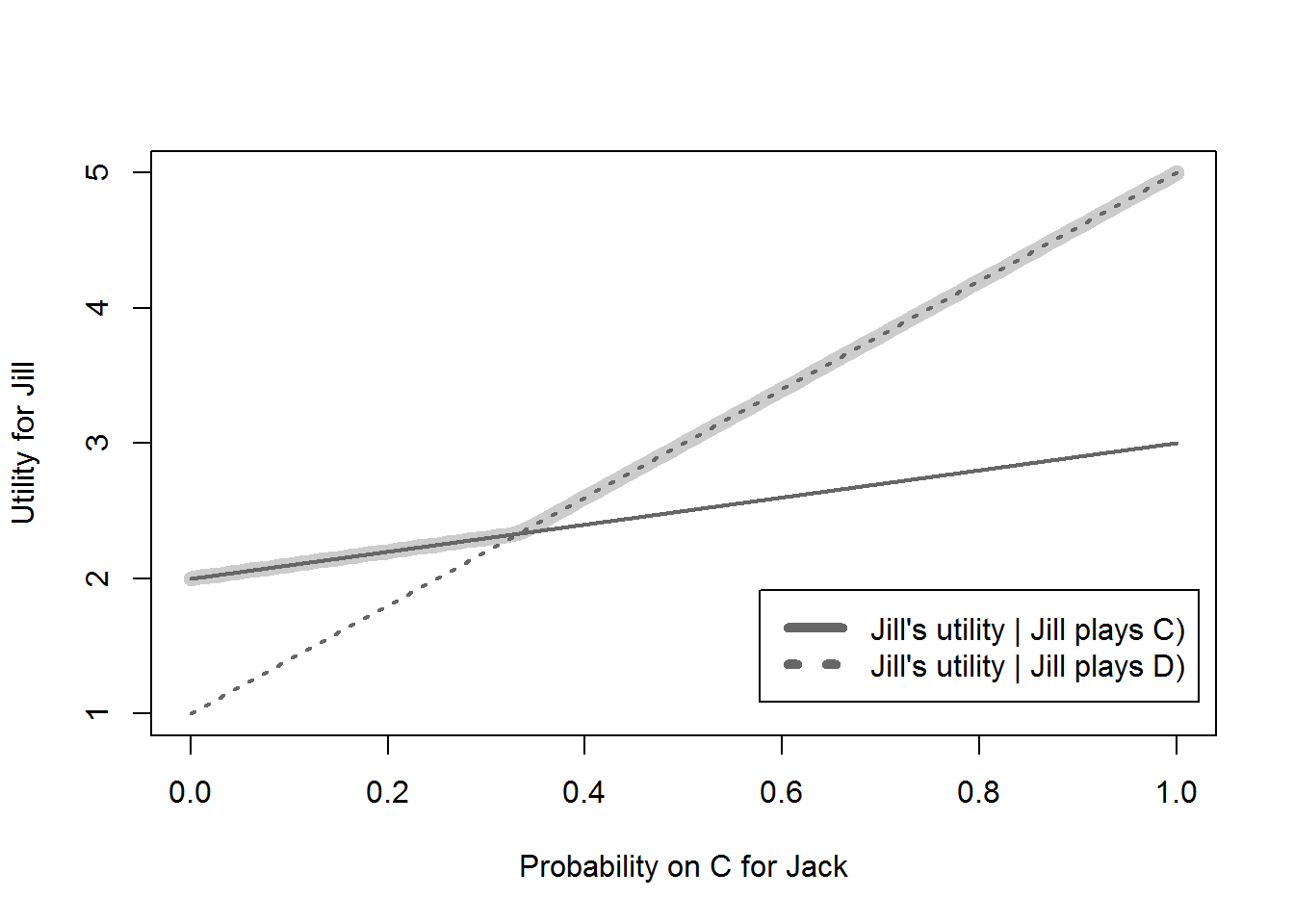From that figure you can also see which action by Jack makes Jill indifferent between her two strategies. Another way to show the best responses is to define the sapce of the graph to be the actions by both players, then you can plot the optimal action by Jill as a function of Jack’s actions directly. This is done using the same function but turning off the `utilities` switch and turning on the `br` (best responses) switch:

``gt_brgraph(Chicken,  P1 = "Jack", P2 = "Jill", br = TRUE, util = FALSE, draw2 = FALSE)``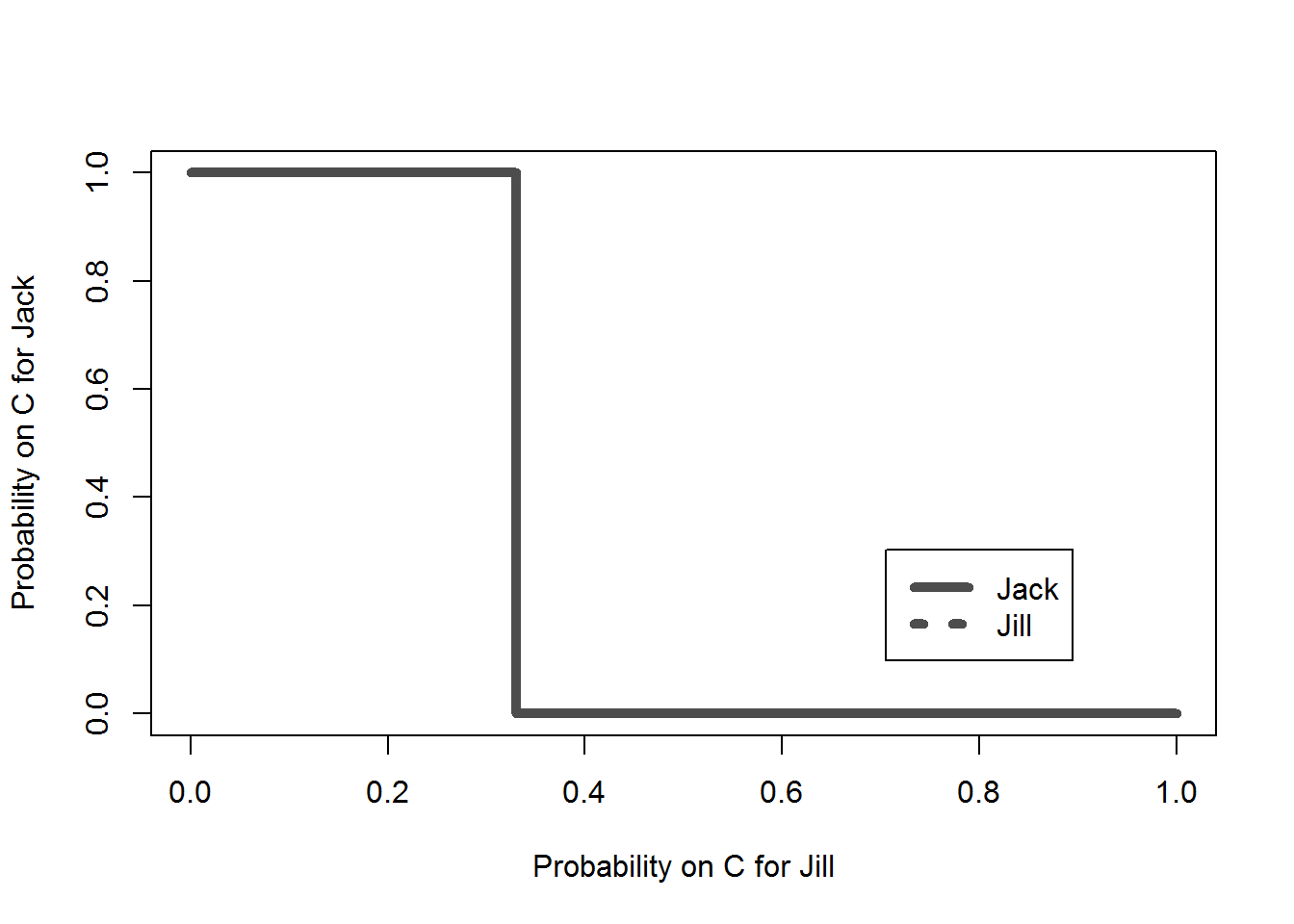You can do the same for Jack:

``gt_brgraph(Chicken,  P1 = "Jack", P2 = "Jill", br = TRUE, util = FALSE, draw1 = FALSE)``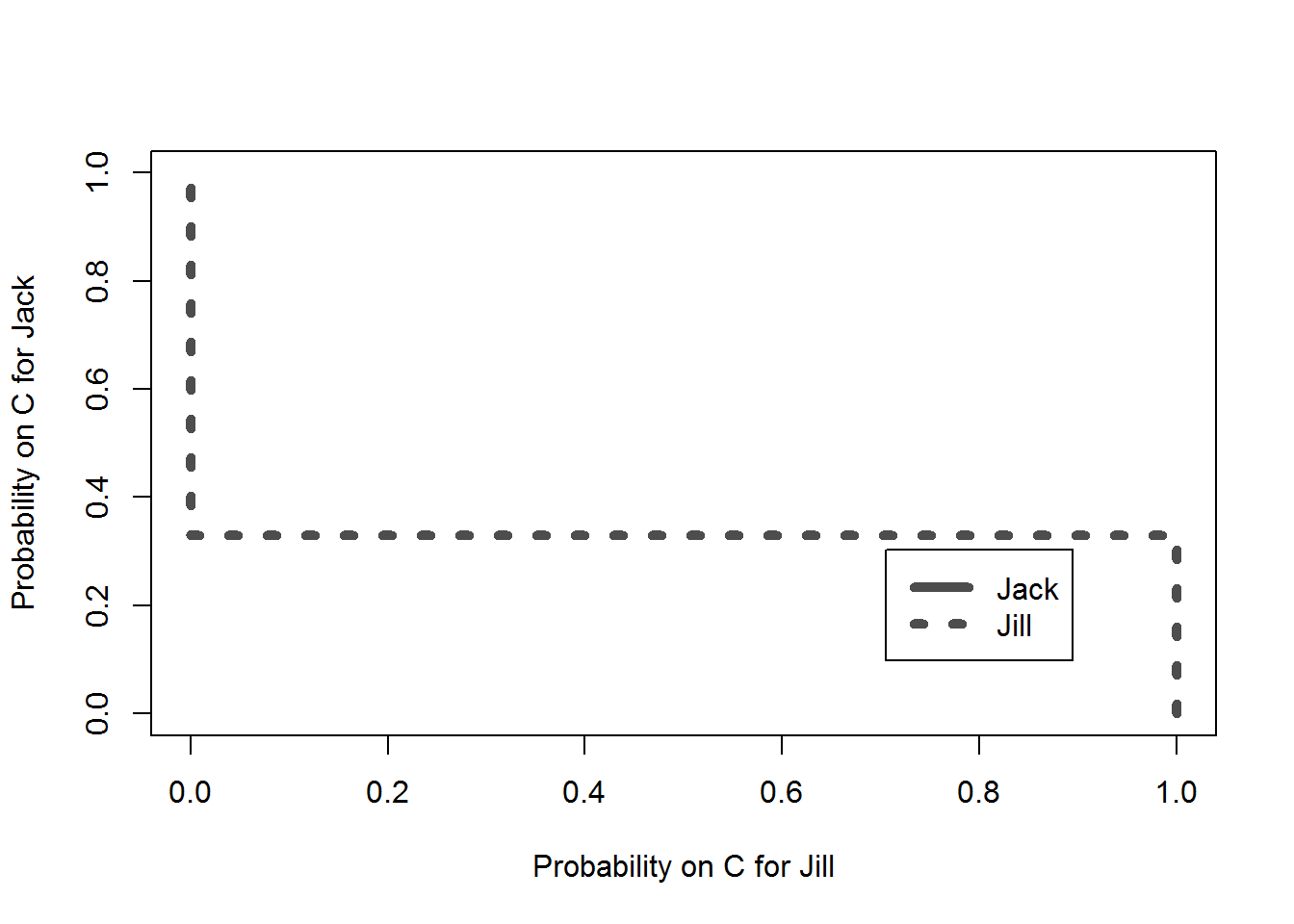And then the intersection is the Nash equilibrium.

``gt_brgraph(Chicken, P1 = "Jack", P2 = "Jill",  br = TRUE, util = FALSE)``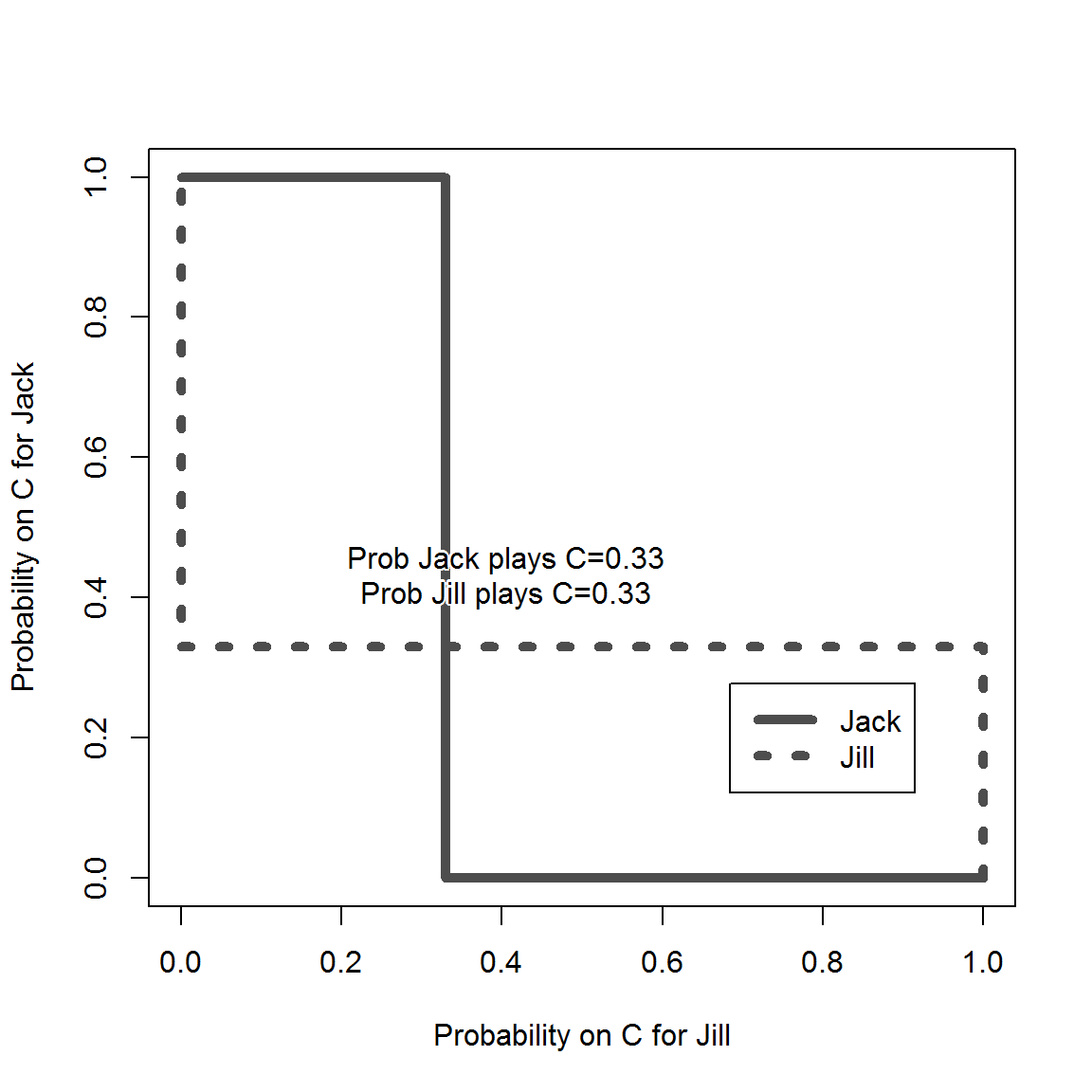## Continuous spaces

The same basic principle applies to games in which people have a continuum of strategies. Here is an example of a second prince sealed bid acution. Anyone can bid between 0 and 400 and each person ends up with the value of the object (if they get it) less the pricec they pay (if they pay). Under the rules the highest bidder wins but pays the price of the second highest bidder. Dun values tehgood at 200 and Dee at 400. The `gt_brcplot` function graphs the best responses (most of the arguments below are for formatting).

``````u1=function(a1,a2){(400-a2)*(a1>a2)+(1/2)*(400-a2)*(a1==a2)+0*(a1<a2)}
u2=function(a1,a2){(200-a1)*(a2>a1)+(1/2)*(200-a1)*(a1==a2)+0*(a2<a1)}
gt_brcplot(u1=u1, u2=u2, player = 3, type="br",
n.grid = 21,  s1=0,s2=1000,
colbg1br = "white", brcex1=3.5,
colbg2br = "red",   brcex2=1.5,
xlab=paste("Dee's bid"), ylab=paste("Dum's bid"))``````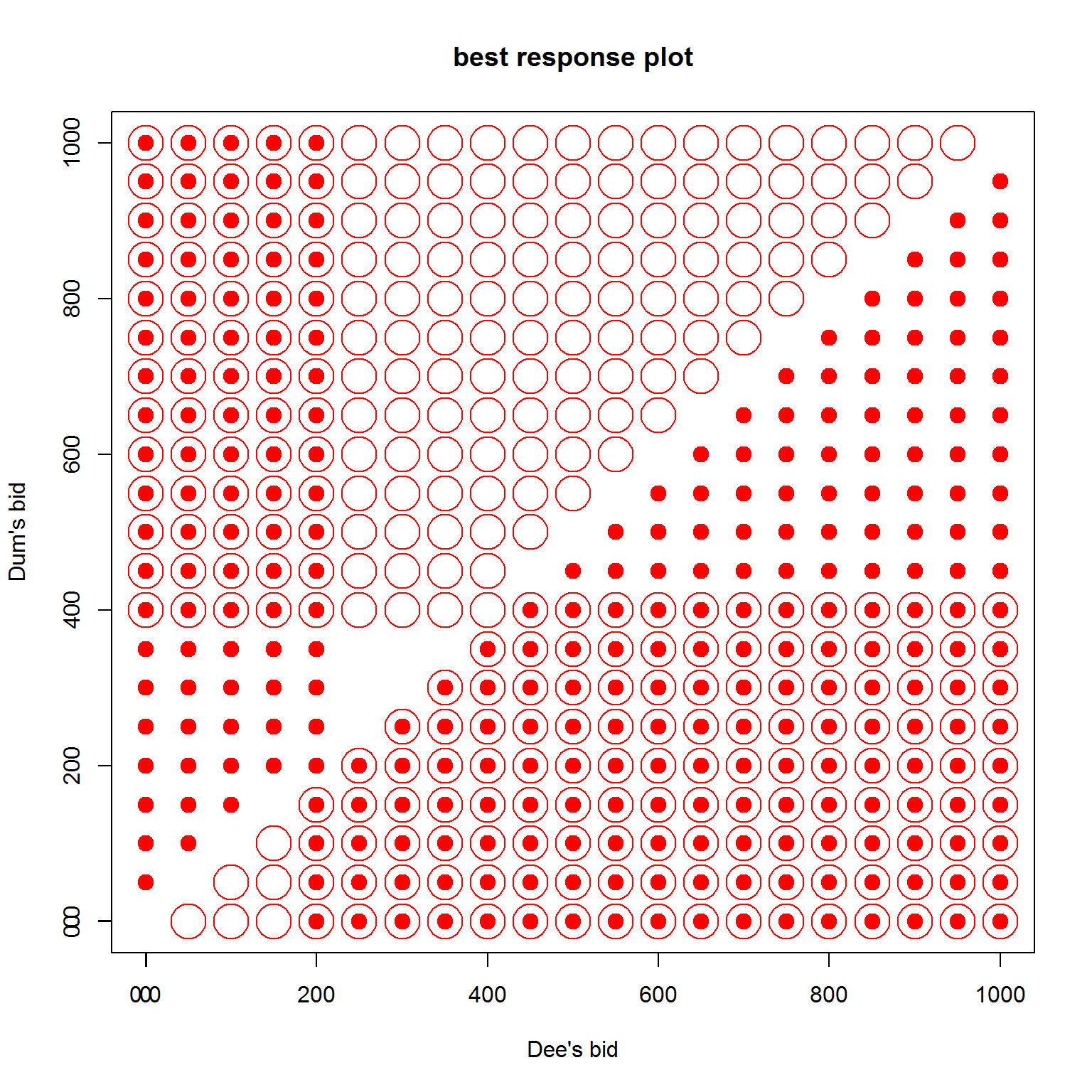Here the solid dots are Dum’s reactions to Dee’s bid. The hollow dots are Dee’s reactions to Dum’s bid. The points with a solid dot inside the hollow dot are all points in which both player’s best responses to an ation are the action itself – in other words these are all nash equilibria.

The same function can represent continuous game in other ways such as using contour plots or surface plots. THe figure below shows continuous reactions to the Dixit-Londregan game described in Political Games.

``````u1=function(a1,a2){.5+.3*a1/(a1+a2)+.15*(1-a1)/(2-a1-a2)}
u2=function(a1,a2){.5+.3*a2/(a1+a2)+.15*(1-a2)/(2-a1-a2)}
gt_brcplot(u1=u1, u2=u2, player = 3, type="br", cont = TRUE,
n.grid = 400, brtype="l", clevels=10, brlwd=2,
tol=.0000005, col1br = "red",
col2br = "black", col1="grey", starfill="red",
xlab="Action by party 1",   ylab = "Action by party 2")``````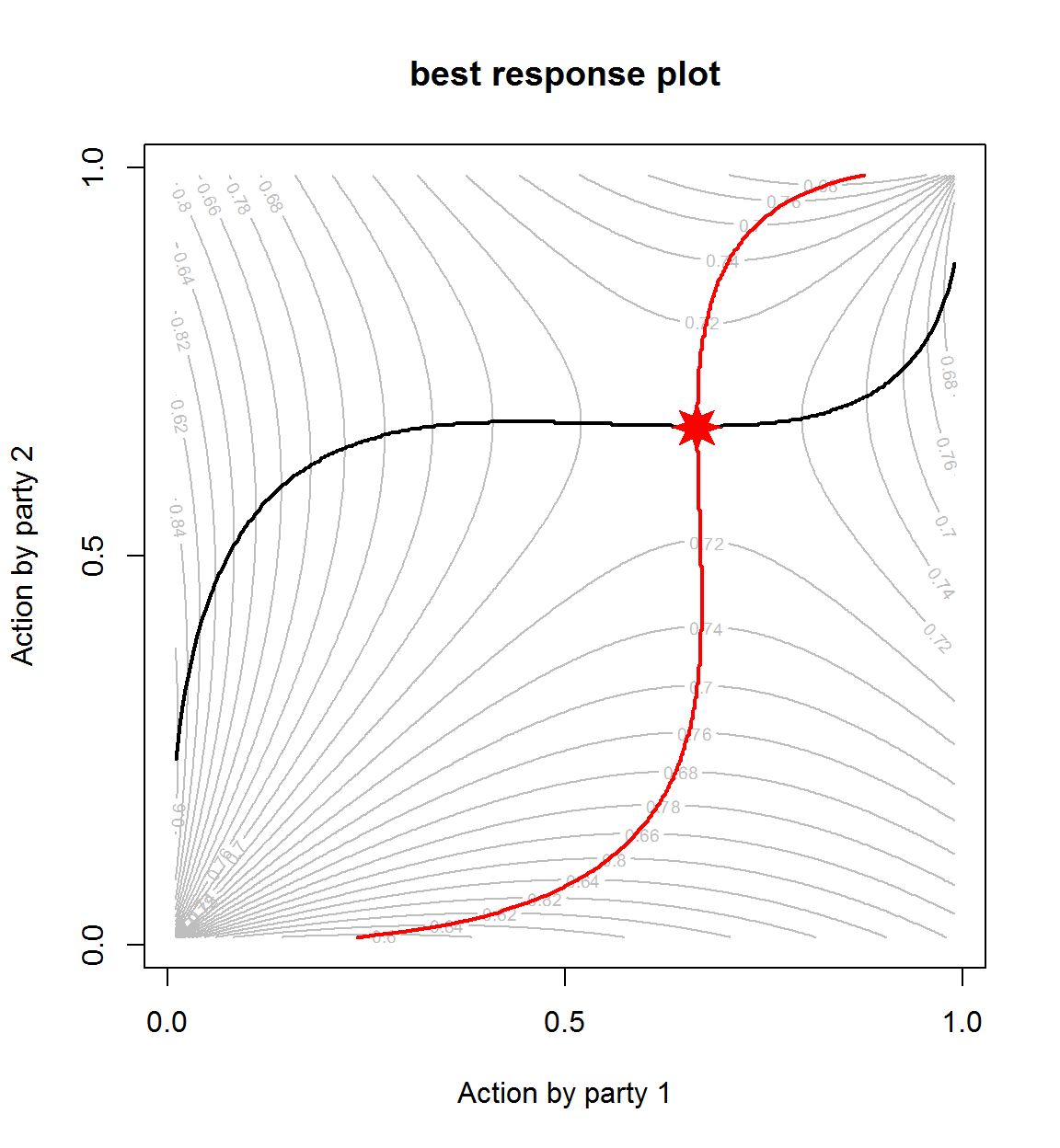## Minimax illustration

Here is an example of a zero sum game like that described in appendix A2.

``````cat_and_mouse <- matrix(-c(0, 1, .75, .25),2)
gt_bimatrix(X = cat_and_mouse, Y = -cat_and_mouse, P1 = "Jill", P2 = "Jack",  labels1 = c("L", "R"))``````